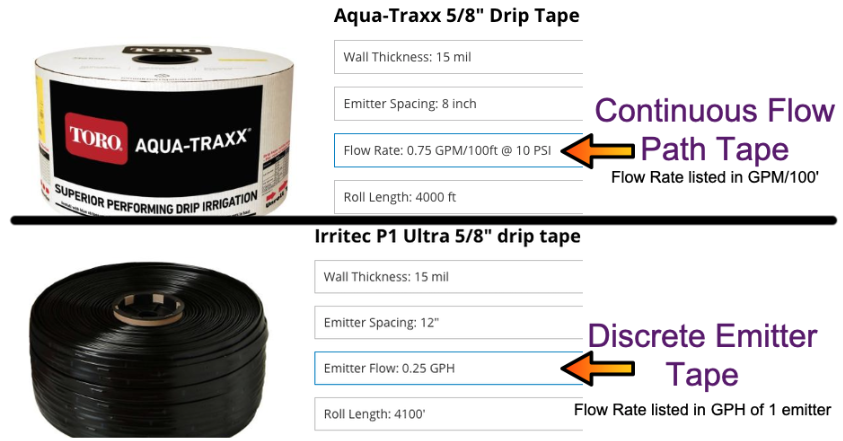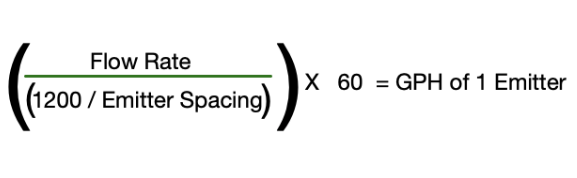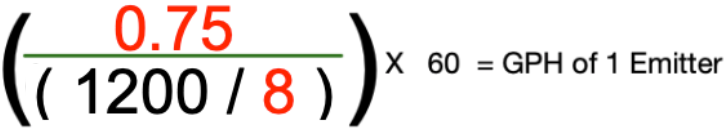Drip tape can be separated into two different types. Continuous Flow Path Tape and Discrete Emitter Tape. The flow rates or emission rates for the two tape types are often listed differently. This can cause confusion as we usually are talking in GPH with drip irrigation.

• Continuous Flow Path Tape is listed in Gallons Per Minute per 100 feet (GPM/100’).

•  Discrete Emitter Tape is often listed in GPH per one emitter.

Note: Dripline tubing is usually listed in GPH per one emitter.

Both Discrete Emitter Tape and Continuous Flow Path Tape are widely used. Below are examples of each type of Tape:How do I find out the flow rate of 1 emitter in GPH if I have Continuous Flow Path Tape listed in GPM/100’ ?

You can use this Formula to convert GPM/100’ to GPH of one emitter:The Flow Rate will be the listed flow rate in GPM/100ft and the Emitter Spacing will be the listed emitter spacing in inches.

Example: Using the Aqua-Traxx ⅝” Drip Tape pictured above we can use the formula to determine the flow rate of one emitter in GPH.

• In the image, we can see the Aqua-Traxx has a Flow Rate of 0.75 GPM/100ft and an Emitter Spacing of 8 inches

• Flow Rate = 0.75 GPM

• Emitter Spacing 8”1. First divide 1200 by 8:         1200/8 = 150

2. Next divide 0.75 by 150:      0.75/150 = 0.005

3. Last multiply 0.005 by 60:    0.005 x 60 = 0.3 GPH

The nominal flow rate of one emitter in GPH for this roll of Aqua-Traxx Drip Tape is 0.3 GPH